# Three digit-two digit subtraction with regrouping tens:grade 2

##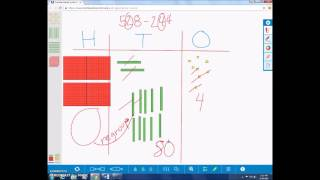By university of Houston mathematics education

The video describes student strategies for three digit subtraction regrouping tens using concrete, pictorial and abstract models.# Three digit-two digit subtraction with regrouping:grade 2

##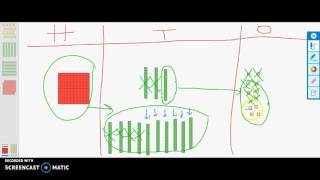By university of Houston mathematics education

The video describes a student strategy for subtraction with regrouping using concrete, pictorial and abstract models.##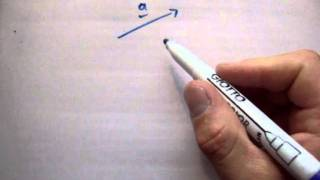By Adam Beatty

YouTube presents Vectors : adding and subtracting vectors, an educational video resource on math.# Three-Digit Subtraction with Counting Strategies: Grade 2

##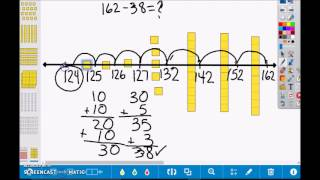By university of Houston mathematics education

The video describes counting strategies using place value to subtract.# Subtraction word problems within 10

##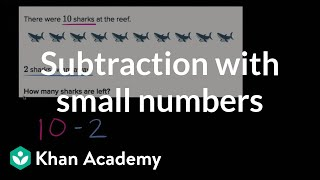By Khan Academy

Learn how to solve word problems by subtracting small numbers (numbers 10 or less).# Subtraction within five

##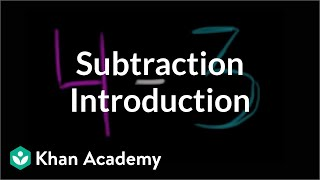By Khan Academy

Learn what it means to subtract. The examples used in this video are 4-3 and 5-2.# Multi-digit Subtraction

##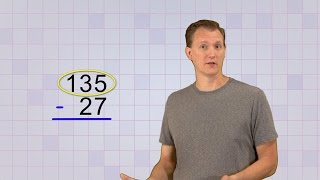By mathantics

Multi-digit Subtraction# Grade 2 Math 2.2, Counting back for Subtraction

##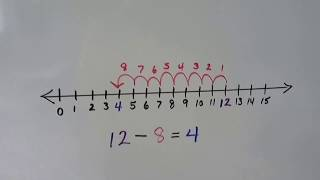By Joann's School

How to count back to subtract by using a number line. Find the difference between two numbers.# Subtraction within 20

##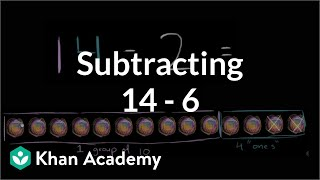By Khan Academy

Learn how to subtract 14 - 6 by first thinking about subtracting 2 and 4.# Subtraction within 100

##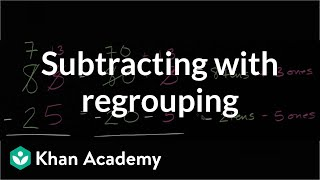By Khan Academy

Learn how to subtract 23 from 65 by thinking about tens and ones.# Adding and Subtracting Complex Numbers

##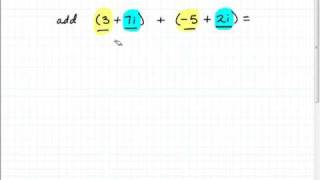By mathonpoint

This video explains how to add or subtract complex numbers. Two example problems are completed in a step-by-step manner.##By Adam Beatty

YouTube presents Vectors : adding and subtracting vectors, an educational video resource on math.# Fraction Subtraction With Borrowing

##By Ron Cox

In this five-minute video the instructor uses humor and computer software to demonstrate how to borrow when subtracting with fractions. This video is a clip from a larger segment. The explanations are clear and easy to understand.# Subtraction

##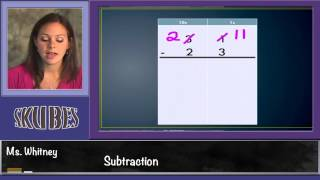By Skubes ed

Fluently add and subtract within 1000 using strategies and algorithms based on place value, properties of operations, and/or the relationship between addition and subtraction.# Subtracting 1 vs. subtracting 10 | Addition and subtraction within 100

##By Khan Academy

Sal talks about the difference between subtracting 1 and subtracting 10.# Addition and subtraction trig identities

##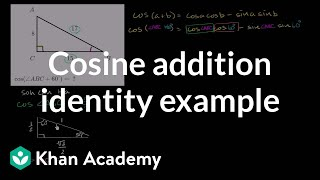By Khan Academy

Sal evaluates the cosine of the sum of 60ï¿½ï¿½ï¿½ï¿½ï¿½ï¿½ï¿½ï¿½ï¿½ï¿½ï¿½ï¿½ and another angle whose right triangle is given. To do this, he must use the cosine angle addition formula.# Addition and subtraction with number lines

##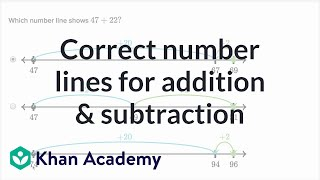By Khan Academy

Sal figures out which number line matches up with a 2-digit addition or subtraction problem.# Addition and subtraction trig identities

##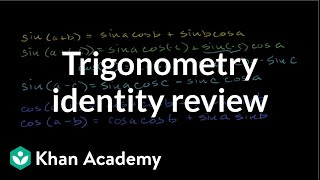By Khan Academy

Sal reviews all the different trigonometric angle addition identities: sin(a+b), sin(a-c), cos(a+b), cos(a-b), cos(2a), and sin(2a).##By Khan Academy

Sal solves missing number in problems like 73 = ___ + 57.##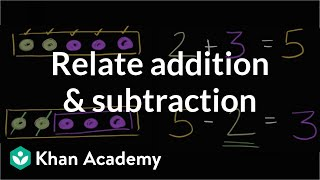By Khan Academy